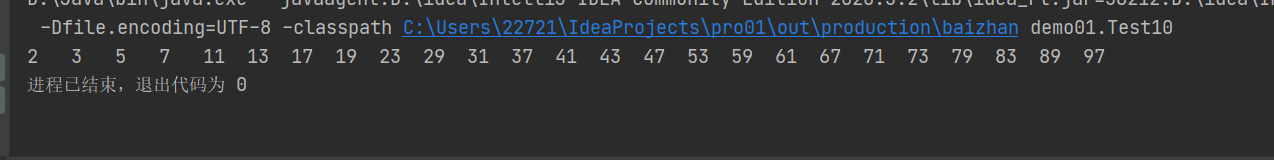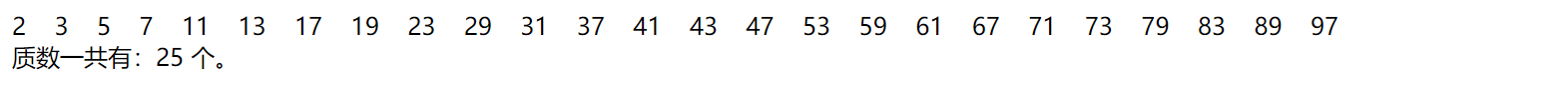• java输出1-100之间的全部素数 public class Test10 { public static void main(String[] args) { int i = 2; while (i <= 100) { int num = 2; boolean flag = true; while (num < i) { if (i % num

java输出1-100之间的全部素数

public class Test10 {
public static void main(String[] args) {
int i = 2;
while (i <= 100) {
int num = 2;
boolean flag = true;
while (num < i) {
if (i % num == 0) {
flag = false;
}
num++;
}
if (flag) {
System.out.print(i+"\t");
}
i++;
}
}
}

执行代码如下：展开全文• 一个大于1的自然数，除了1和它自身外，不能被其他自然数整除的数叫做质数；否则称为合数（规定1既不是质数也不是合数）。 判断一个数是否是素数 public class java_4 { public static void main(String[] args) { ...

学习目标：

目标：熟练运用 Java所学知识

题目内容：

本文内容：判断一个数是不是素数，输出1-100之间所有素数

实现思想：

素数的定义：

质数又称素数。一个大于1的自然数，除了1和它自身外，不能被其他自然数整除的数叫做质数；否则称为合数（规定1既不是质数也不是合数）。

思路：

1.素数除了1和他本身外不能被其他数整除
2.所以使用一个小于当前数并且大于一的循环for(int i=num-1;i>1;i- -)，其中如果有能够整除当前数的值则不是素数，否则是素数

实现代码：

判断一个数是否是素数

public class java_4 {
public static void main(String[] args) {
//判断一个数是否是素数
System.out.print("请输入一个整数：");
Scanner scanner=new Scanner(System.in);
int num=scanner.nextInt();
System.out.println(num+"是一个素数");
}
else{
System.out.println(num+"不是一个素数");
}
}
private static boolean isPrimeNum(int num) {
for(int i=num-1;i>1;i--){
if(num%i==0)
return false;
}
return true;
}
}

输出1-100之间所有素数

public class java_4 {
public static void main(String[] args) {
for(int i=1;i<101;i++){
if(i==1) {
System.out.println("1不是素数。也不是合数");
System.out.printf("%d是素数\n",i);
}
}
private static boolean isPrimeNum(int num) {
for(int i=num-1;i>1;i--){
if(num%i==0)
return false;
}
return true;
}
}

展开全文java
• 代码如下： <!DOCTYPE html> <html lang="zh"> <head> <meta charset="UTF-8" /> <meta name="viewport" content="width=device-width, initial-scale=1.0" />...Doc

代码如下：

<!DOCTYPE html>
<html lang="zh">
<meta charset="UTF-8" />
<meta name="viewport" content="width=device-width, initial-scale=1.0" />
<meta http-equiv="X-UA-Compatible" content="ie=edge" />
<title>Document</title>
<script type="text/javascript">
var sum = 0;
for(var i = 1;i <= 100;i++){
var flag = true;

if(i == 1)
flag = false;
for(var j = 2;j < i;j++){

if(i % j == 0){
flag = false;
break;
}
}
if(flag){
document.write(i + "&nbsp;&nbsp;&nbsp;&nbsp;");
sum++;
}
}
document.write("<br />质数一共有：" + sum +  " 个。");
</script>
<body>

</body>
</html>

运行结果如下：展开全文javascript js
• int main() { int n,m; scanf("%d",&n); for(m>2;m<n;m<n) if(n%m==0)break; if(n==m) printf("是");...//打印1-100素数； /* int ss(int m); int main() { int m; for(m=0;m...

int main()
{
int n,m;
scanf("%d",&n);
for(m=2;m<n;m++)
if(n%m==0)break;

if(n==m)
printf("是");
else
printf("否");

return 0;
}
*/
//打印1-100的素数；
/*
int ss(int m);
int main()
{
int m;
for(m=0;m<100;m++)
if(ss(m))
printf("%d ",m);

}

int ss(int m)
{
int n;
for(n=2;n<m;n++)//将n赋值为2 利用素数的性质
if(m%n==0)break;//表示1之外除了他自己本身没有数能被整除

if(m==n)
return 1;
else
return 0;
}*/

展开全文c语言
• for(int i=100;i<=200;i++){ for(int j=2;j<=i;j++){ if(i%j==0){ break; } else{ count++; printf(" %d",i); if(count%8==0){ printf("\n"); } break; ...蓝桥杯 c语言
• 一、写一个函数，判断1-100之间有多少个素数输出所有素数？function panduan($number = 100){$sumCount = 0; // 输出次数for($i = 1;$i < $number;$i++){$count = 0;// 整除次数for ($j = 1; $j <=$i; $j... •c语言 • C语言，编写函数判断一个整数是否为素数，在主函数中调用该函数并输出100以内的全部素数？参考代码：#include &ltstdio.h&gtint is_prime_number(int n){int iint result = 1if(n==1)return 0for(i=2i&... • 6.输出1-100中所有的素数 *素数也称质数。一个大于1的正整数，如果除了1和它本身以外，不能被其他正整数整除，就叫素数。如2，3，5，7，11，13，17… /* * 6.输出1-100中所有的素数 *素数也称质数。一个大于1的正...java • i=2 #1-100第一个素数是2 for i in range(2,100): for j in range(2,i): if i%j==0: #在2到（i-1）中找是否能找到一个数能被i整除 break #不能的话就退出内层循环 if i%j!=0: print(i) #逐个输出素数 ...python • 作为一个python初学者的闲暇之余作品 from __future__ import print_function for i in range(2,101): t=0 for j in range(2,i): if i%j==0: t=1 if t==0: print(i,end=' ') 水的不能在水……python • #include<stdio.h>...=100;i++) { for(j=2;j<=sqrt(i*1.0);j++) { if(i%j == 0) { break; //跳出一层循环 } } //break跳到这 if(j > sqrt(i*1.0)) { printf • 素数(质数)：只能被1和它本身整除的数。 注：1不是素数 思路：问题就在于如果是素数就要不断的去试除来证明，直到全部排除； 1： #include<stdio.h> int main() { int i = 0; int j = 0; for(i = 100; i &...c语言 • 使用Java求出1-100之间素数之和，其实其他语言也差不多，只是思路算法的问题。 首先： 素数：只能被1和其本身整除的数 也就是在1和x本身之间，只要能被1–x之间的数整除就不是素数 所以： 循环遍历1-100个数x，每...java 数据结构 算法 • 打印1-100之间所有的质数 质数是指在大于1的自然数中，除了1和它本身以外不再有其他因数的自然数。 具体思路： 首先需要找到1-100之间所有的数 判断是否为质数 打印出找到的质数 <script> // 打印出1-100...javascript • public class forprime {public static void main(string[] args) {int sum = 0;... 100; i++) {if (isprime((long) i)){system.out.println(" isprime = " + i);sum = sum +i;}}system.out.prin... • 1】题目：C语言编写输出100~200之间素数 【2】程序设计如下： #include <stdio.h> int main() { int prime(int n); int i, j = 0; for( i = 100; i <= 200; i++ ) { if(prime(i)) { printf("%3d...c语言 素数筛 • #include <stdio.h> #include <math.h> int main() { int i,n,c,t; for(i=200;i<=200;i++) { c=0; n=sqrt(i); for(t=2;t<n;t++) { if(i%t0) c++; break;//提前结束本次循环，提高...... • java中怎样判断一个数是否为素数并且输出1-100间的素数 ** 怎样判断一个素数 素数的定义：只能被1和本身整除 代码如下： public class Test{ public static void main(String[] args) { int a=4; int b=11; System....java 算法 • 这个代码很巧妙，个人的理解都写...河南科技学院学报(自然科学版),2013,(6):61-64.DOI:10.3969/j.issn.1008-7516.2013.06.015.//输出100以内的素数,思路：//判断素数方法1：//假如自然数N不是素数，则除1和其本身之... • 统计100~200之间素数的个数并输出素数 分析： 素数是指除了 1 和自己本身能被整除外，不能被任何其他的数整除的数； 同时，1 既不是素数也不是合数，2是最小 的合数； Ⅱ、实现过程如下： 1、运行软件VScode，亲测可...javascript html 前端 • //求100以内质数for ($i = 1; $i <= 100;$i++) {$k = 0;for ($j = 1; $j <$i; $j++) {if ($i % $j == 0) {$k++;}}if ($k == 1) {echo$i;echo "";}}?>拓展内容phpPHP(外文名:PHP: Hypertext Preprocessor...
• 该楼层疑似违规已被系统折叠隐藏此楼查看此楼public class PrimeNumber {public static void main(String[] args) {final int NUMBER_FOR_PRIMES = 40;... // 声明每行输出多少素数int count = 0; //...java循环输出质数
• 用python实现1-100之间的质数

千次阅读 2021-11-16 19:51:31
for i in range(2, 100): f = True for j in range(2, i): if i % j == 0: f = False break if f: print(i)selenium 测试工具 python
• python输出2到100之间的素数

千次阅读 2021-03-17 12:32:12
目的：计算2-100之间素数的个数，返回结果什么是素数？素数是指在大于1的自然数中，除了1和它本身以外不再有其他因数的自然数。代码实现：# 定义一个列表接受返回的质数list_num = []def primeNum(n, m):# 遍历n-m...
• public static void main(String[] args) { //一个大于1的自然数，除了1和它自身外，不能被其他自然数整除的数叫做质数素数 for(int i=2;...=i-1;j++) { if(i%j==0) {//质数只能被1或自身整除 ...html 算法 机器学习
• // 第一种方法 function isPrimeNumber(n) { // 除了了1和他自身外 所以从2开始并不包括n for (var i = 2; i < n; i++) { // 如果找到了因子 (%i得0) if (n % i === 0) { // 立即判断出不是素数 ...html5 前端
• //100以内的所有质数 //一般从2开始 //质数：只能被1和自身整除 //定义一个变量或者一个开关 var arr=[]; //第一步：获得所有2-100之间的数字 for(var i=2;i<=100;i++){ arr.push(i); //再次遍历，拿到2-i...javascript js
• import java.util.Scanner; public class Main { public static void main(String[] args) { Scanner x=new Scanner(System.in); int size=x.nextInt();... int number=1; for(int i=1;i<siz.java...